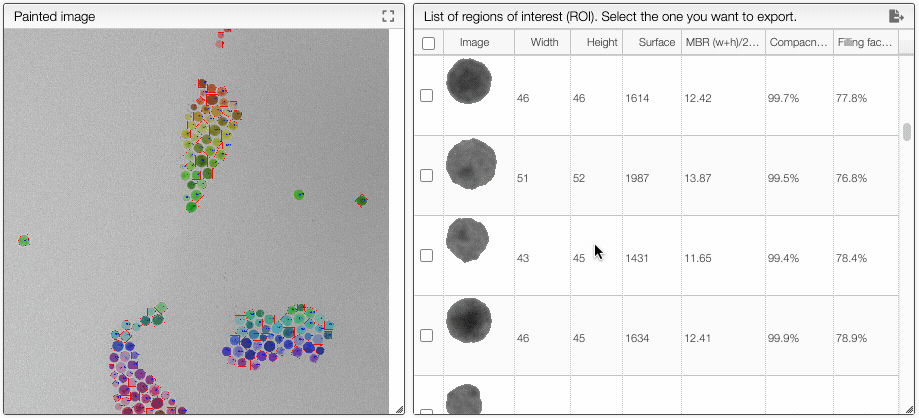# Image analysis

This view allows to annotate images as well as identify Region of Interests on grey scale images.

If the source if a color image a first step will be to convert it to a grey scale image using one of the provided algorithms.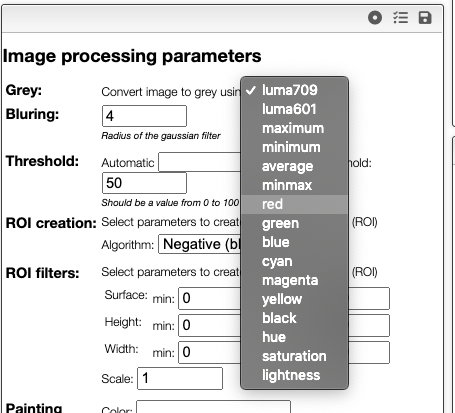If you don't know which algorithm to use you may Explore greys. The system will reduce the size of the image and try all the available algorithms. You should then select the one that yields to the highest contrast.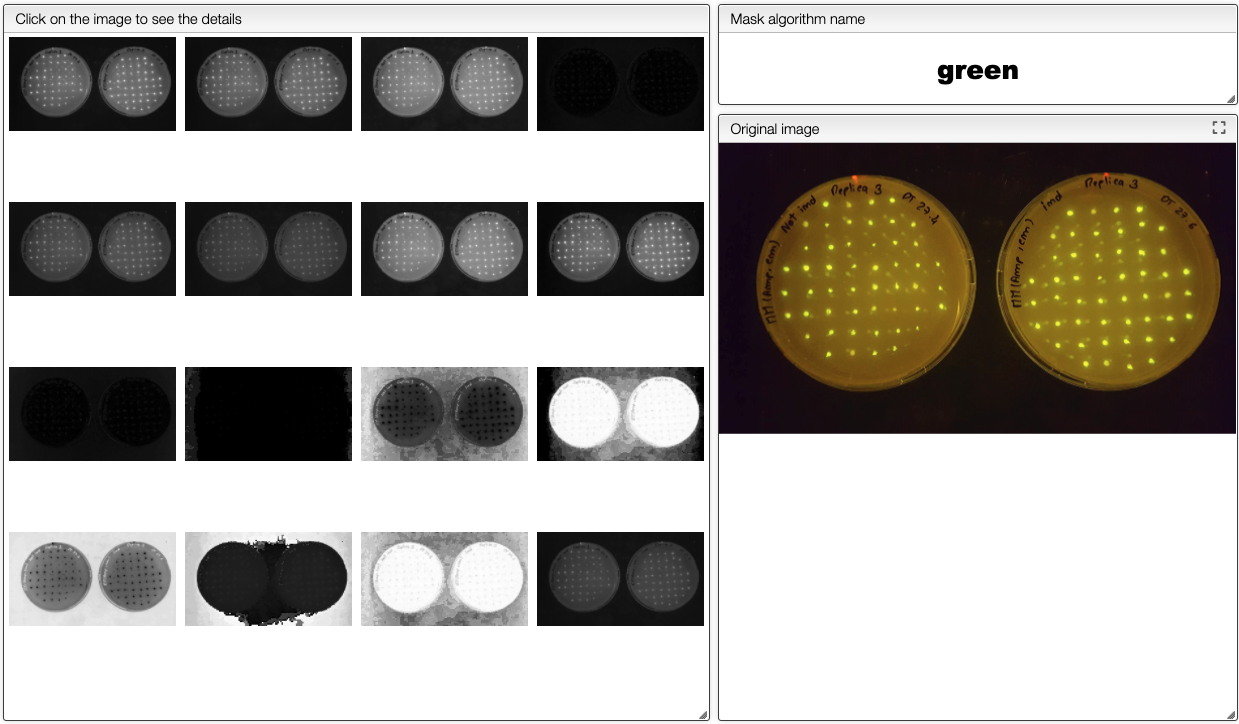## Example of Analysis of SEM / TEM images​

The image coming out of the SEM microscope is expected to be in TIFF 16 bits. Apparently some software destroy the image to 8 bits when adding the scale.

There are 3 steps in order to process your image:

1. The first step is to apply a gaussian filter to the image (blurring). Values of 4 to 8 seem reasonable.
2. The second step will generate a mask. The white pixels are the 'positive' area while the black one negative. You may either select one of the provided algorithms or use the 'threshold' algorithm. In this later case the 'Threshold for mask' value will be taken into account. It should be a value between 0 and 100 (percent of white).
3. Finally the third step is to create the Regions Of Interest (ROI). It is possible to define the minimal surface, positive / negative as well as the 'Scale' factor that will be applied to the ROI.

In order to create regions of interest (ROIs) it is required to create a mask (a black and white image). Creation of the mask is based on the threshold that define the level of grey intensity that separates the white pixels from the black pixels.

This threshold can be determined automatically using one of the provided algorithm or it can be entered manually as a value between 0 and 100% if the algorithm stays unspecified.

In order to quickly evaluate which algorithms or threshold should be used you can click on Explore masks and select the more suitable threshold. This function requires that blurring has been applied before.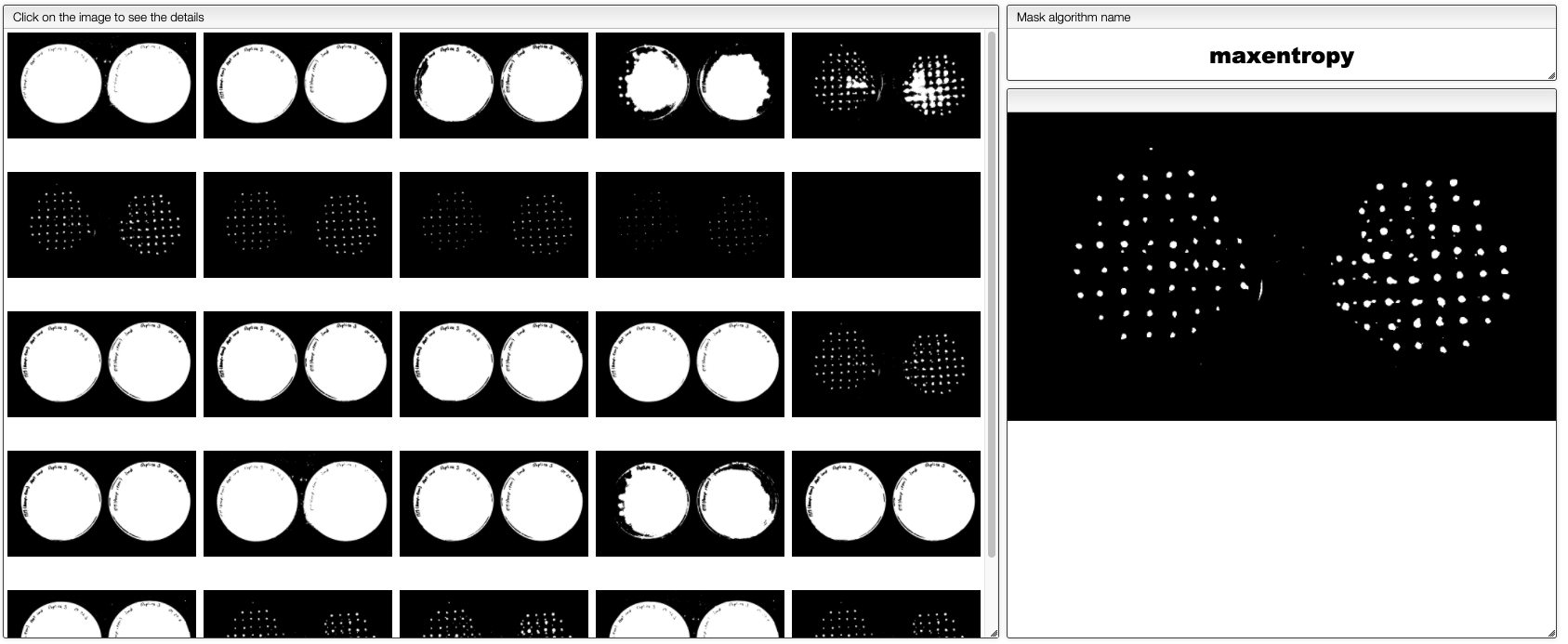### Creating and filtering ROIs​

Once the threshold has been determined it is time to create the ROIs. 4 algorithms are available:

• Positive: the ROIs are white
• Negative: the ROIs are black
• Watershed: there are some ROIs that overlaps and we need to separate them. The ROIs are white
• Inverted watershed: same as Watershed but the ROIs are black

There is also the possibility to scale the obtained ROIs. This may be practical in order to get average color of a spot.

### Painting the final image​

The result of analysis can be graphically displayed on the original image and many options allow defining what should be displayed.

### ROI Analysis​

By clicking on the ‘ROI Analysis’ button you will have access to a table containing various parameters of the different ROIs.

Among other you will have access to the MBR (Minimal bounding rectangle). The ratio between the surface of the MBR and the surface of the ROI (Region Of Interest) is what we call the ‘filling factor’, 100% means a cube, a sphere should be π/4 (78.5%)

### ROI Categories​

If you click on ROI Categories you will be able to define categories based on characteristics of the ROIs. This panel will also display an histogram that contains the different categories.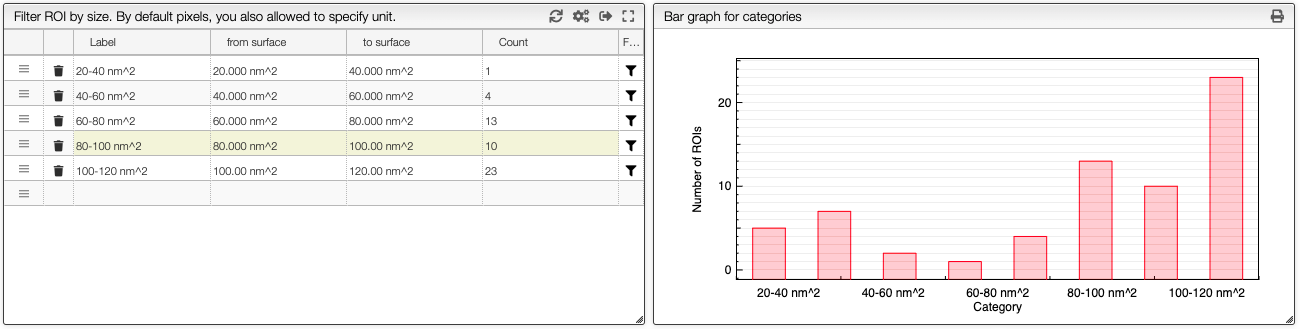Categories
How to create ROI categories.

# Create ROI categories

Region Of Interests (ROIs) can be grouped and counted in categories by clicking on the button ROI categories.

The criteria related to surface or length it is either possible to specify the minimal / maximal number of pixels or to specify the size with the unit.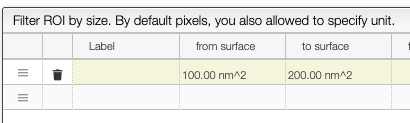You may define an unlimited number of categories based on the available criteria. There is always a from / to range for each of the criteria.

## Checking a specific category​

It is possible to display the ROIs belonging to a specific category by clicking on the filter icon. To come back to the full list of ROIs you should click on the sync icon.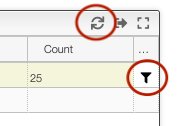From the 3 icons present on the top of the preferences window it is possible to:

• save the current preferences

This allows to store templates of preferences depending the kind of images you are processing.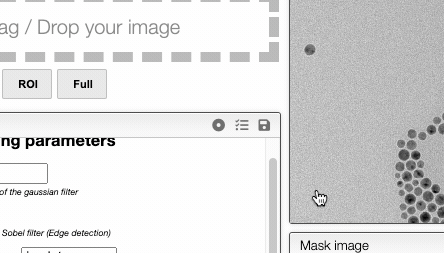### Exporting data and table customization​

It is possible to define the column you want to display / export as well as the format
Columns can be customized by clicking on the icon that is present in the top of the table. Those preferences are used to customize not only which information is displayed but also how it will be exported. This means that if the units are displayed they will also be exported.

If you which to hide the units for importation there is the possibility to specifiy numeral: '0.00', hideUnit: true.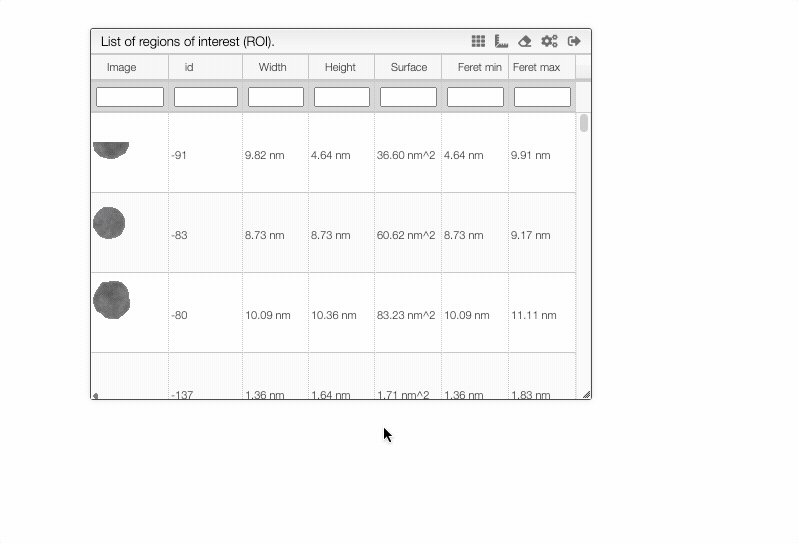### Other tips​

Annotations
It is possible to annotate and measure images with lines and polygons.

# Define pixel size

The image analysis tool allows to draw lines and polygons directly on the image.

All the annotations will be saved with the sample back in the database.

Using annotations it is possible to determine the surface or length of some specific area of the image.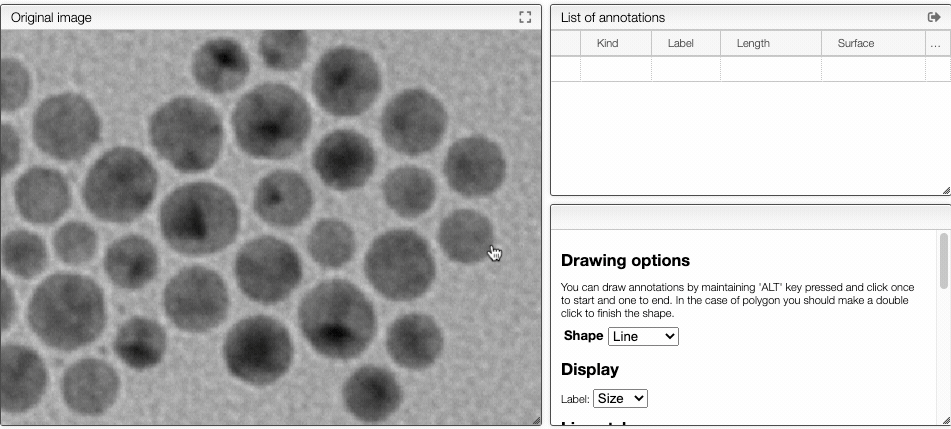## Drawing annotations​

In order to draw annotations:

• for a line: click once for the beginning of the line and another time for the end of the line
• for a polygon: click once fpr aéé the corners of the polygon, click twice for the end of the shape.

## Display labels​

It is possible to display labels either as a custom text or as the value of the shape (length or surface.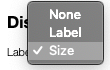## Changing style​

Style of the label and the shape can be customized according to the preferences. Opacity is a value between 0 and 1. 0 meaning fully transparent and 1 fully opaque.

REGIONS OF INTEREST (ROIS)
Many parameters are calculated for regions of interest.

Many parameters characterizing ROIs are automatically calculated.

## ROI​

Region Of Interest (ROI) posses various characteristics. Among them:

• Surface: the number of pixels in the ROI
• External: the number of pixels that touch at least one other external pixel
• Perimeter: ROI perimeter using cutting edge algorithm (see illustration)
• Diameter of a circle of equal projection area (DEQPC): $D_{EQPC} = 2 \sqrt{\frac{Surface}{\pi}}$
• Diameter of a circle of equal perimeter (DPED): $D_{PED} = \frac{External}{\pi}$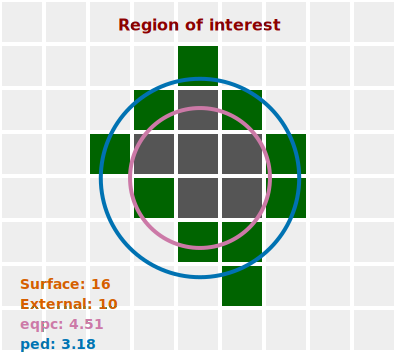Calculation of perimeter of pixel based images is not a trivial topic. This has been reviewed in the literature.

In this tool we use the following approach:

• We count all the pixel sides that are outside the ROI (each side counts as 1)
• If a pixel has 2 external sides, we remove from the sum (2 - √2) = ~0.59
• If a pixel has 3 external sides, we remove from the sum 2 * (2 - √2) = ~ 1.17

This means that for each border pixel the contribution to the perimeter is:

• pixels with one external side: 1
• pixels with two external sides: ~1.41
• pixels with three external sides: ~1.83

Practically the shape of the perimeter is represented in the following image: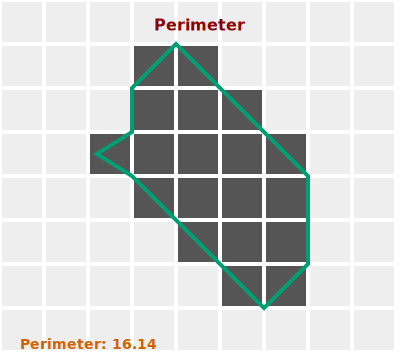## Minimum bounding rectangle (MBR)​

An information that allows to determine the shape of a ROI is the Minimum Bounding Rectange(MBR) that represents the smallest rectangle that surround the ROI.

The MBR is characterized by:

• width: length of the longest side of the rectangle
• height: length of the smallest side of the rectangle
• perimeter: perimeter of the rectangle
• surface: surface of the rectangle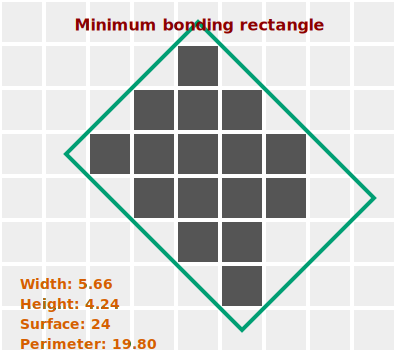## Convex Hull (CH)​

The convex hull may be visualized as the shape enclosed by a rubber band stretched around the ROI. It is useful to estimate the compactness of the ROI.

Two parameters are available for the convex hull:

• surface
• perimeter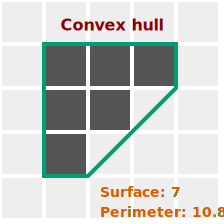## Feret diameters​

The feret diameters of a ROI can be calculated using a virtual caliper. 2 diameters are calculated:

• min: the minimal size of the ROI that could be measured using a caliper
• max: the maximal size of the ROI that could be measured by a caliper
• ψA (Aspect ratio): ratio of the minimum to the maximum Feret diameter (0 < ψA ≤ 1), an indication of the elongation of the particle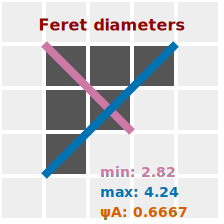## Other parameters​

### Sphericity​

The sphericity is the ratio of the perimeter of the equivalent circle, PPED, to the real perimeter, Preal. A perfect sphere as a sphericity of 1. The idea is that irregular shape causes an increase in the perimeter and therefore this ratio has a lower value. Knowing that the smallest possible perimeter for a given projection area is a circle, 0 < S ≤ 1.

Please note that the evaluation of perimeter is a complex topic and check above the approach that is used in this application. It is not impossible that the sphericity is slightly higher than 1.

$S = \frac{P_{EQPC}}{P_{real}}$

### Convexity​

The convexity ($\psi_C$) describes the compactness of a particle. The convexity is the ratio of the projection area itself and the area of the convex hull. The maximum theoretical convexity is 1, if there are no concave regions. Due to the pixelation of images, all particles seem to have small concave regions, corresponding to the tiny steps with every pixel in the perimeter line. Therefore, the maximum convexity calculated in reality is mostly limited to 0.99.

$\psi_C = \frac{ROI_{surface}}{CH_{surface}}$

### Roundness​

$Roundness = \frac{4 \cdot ROI_{surface}}{π \cdot Feret_{max}}$
Define pixel size
Pixel size can be defined manually or automatically.

# Define pixel size

In order to know the size of ROIs or annotations it is important to define the pixel size.

In some case this information can be extracted from the meta information of the image. It can also be defined by the user using one of the 3 following approaches:

## From the list of images​

In the list of images you can directly double click on the pixel size and enter a value with the corresponding unit.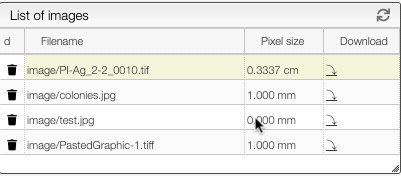## From the annotations tab​

In the annotation tab you can click on the edit button on the top of the Pixel size box.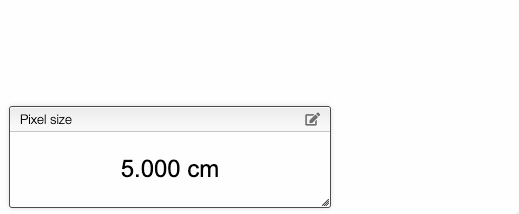You can also draw a line and specify the length of the line. This will calculate automatically the size of a pixel.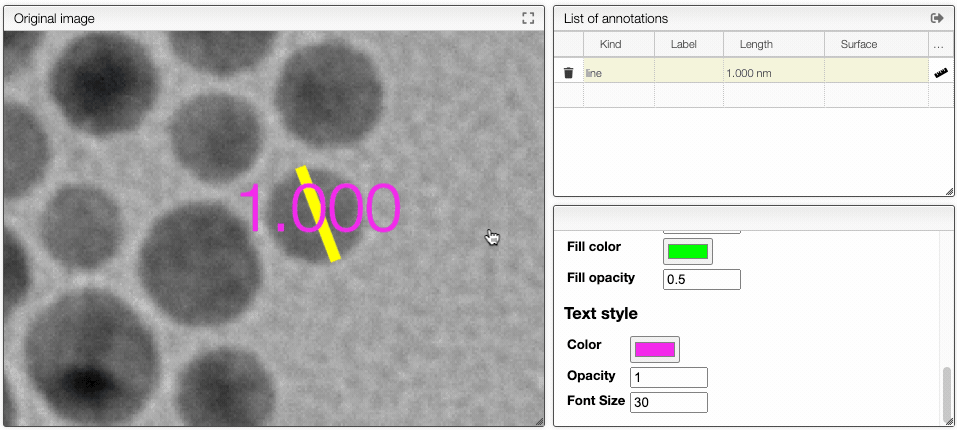Select ROIs
Regions of interest may be selected before exportation.

# Select ROIs for exportation

It is possible to select some region of interests (ROIs) to export either from the ROIs table by clicking the checkbox in the first column or by clicking on the ROI directly in the image.